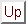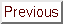Next: The parallel extension of Up: The CONDOR unconstrained algorithm. Previous: The bound .   Contents

# Note about the validity check.

When the computation for the currentis complete, we check the model (see step 14 of the algorithm) around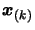, then one or both of the conditions: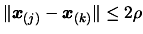(6.5)(6.6)

must hold for every points in the dataset. Whenis reduced by formula 6.4, the equation 6.5 is very often NOT verified. Only Equation 6.6, prevents the algorithm from sampling the model at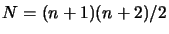new points. Numerical experiments indicate that the algorithm is highly successful in that it computes less then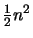new points in most cases.

Frank Vanden Berghen 2004-04-19